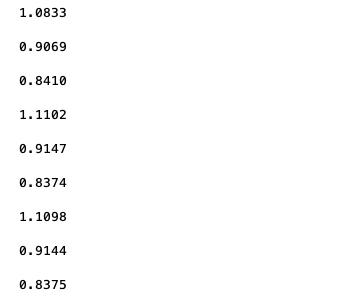# How can I transform this to a "minimize" question?

This is the code:

``````         cvx_begin sdp quiet
cvx_solver sedumi
variable W(M,M) complex semidefinite
maximize real(trace(W*Hw))
subject to
real(trace(W)) <= Pj;
real(trace(W*HRR))+(((norm(diag(v')))^2)*delta1) <= Pi;%xiugai^2
real(trace(W*Hw))+(((norm(hRI(:,m)'*diag(v')))^2)*delta1) <= e;%xiugai^2

cvx_end
``````

Is there a way to change it? Or say no?

`minimize -real(trace(W*Hw))`

Then negate the optimal objective value.

That;s a standard “trick” if using a solver or optimization modeling system which only performs minimization. But it’s not necessary in CVX, because CVX allows minimization or maximization (or neither, for a feasibility problem).

Oh! I see. Let me try it. Thanks, Mark.

Hello Dr. Mark! Now, I have another problem.
I used the Dinklebach to do this optimization problem. Sometimes the data I export will become a loop of some data (Not usually). I wonder that it is the problem of the algorithm i used or something else. In most cases, it is still a case of descending convergence.

like this：Are you solving a CVX problem within each iteration of the Dinklebach algorithm? I am not very familiar with that algorithm, so don’t know what behavior its sequence of iterates should or should not have.

Perhaps you could make inquiries on the behavior of that algorithm at https://or.stackexchange.com/ .If you do so, you should probably be more explicit about the optimization problem you are trying to solve, and what the subproblems and overall algorithm flow are.

I got it! Thanks, Mark!